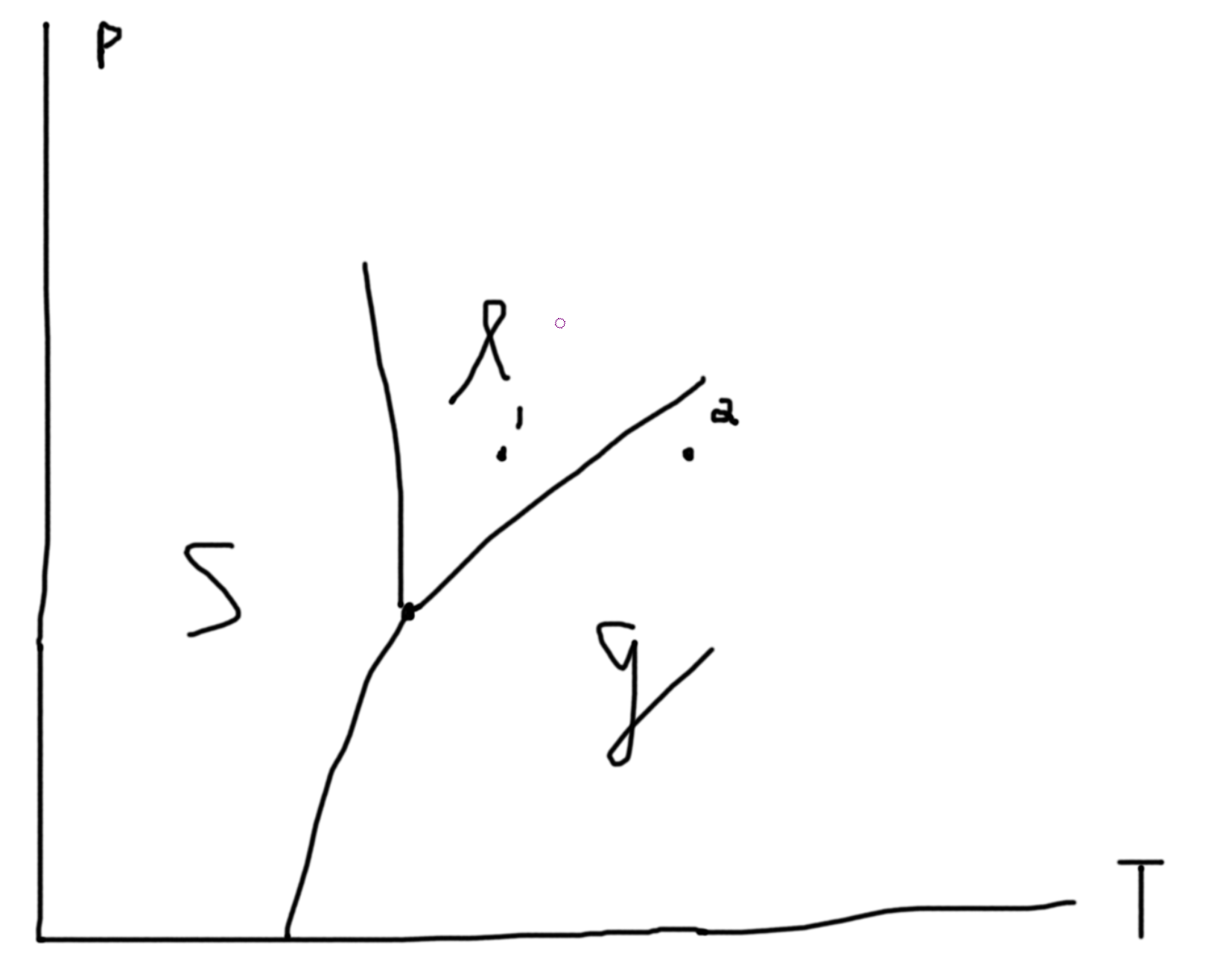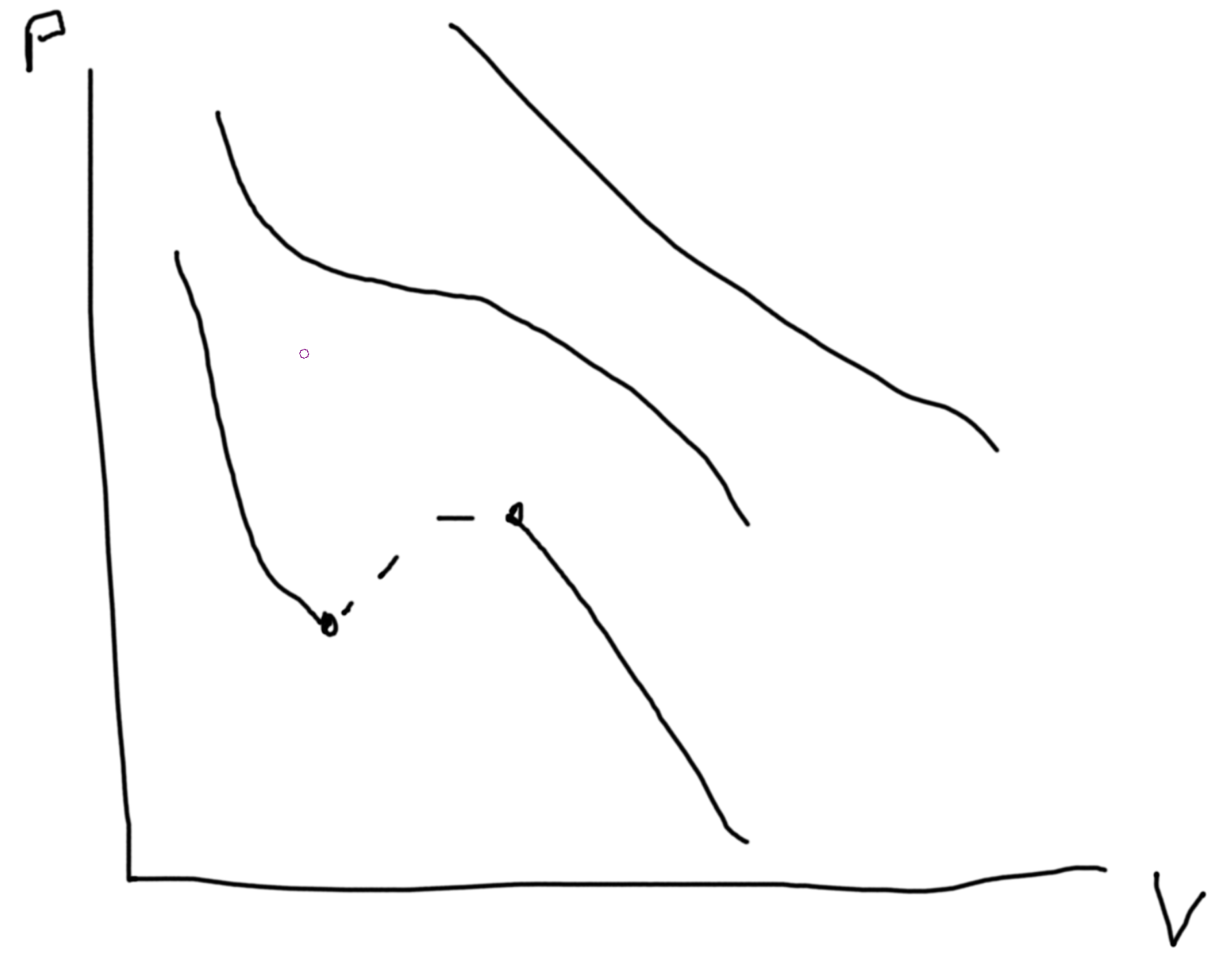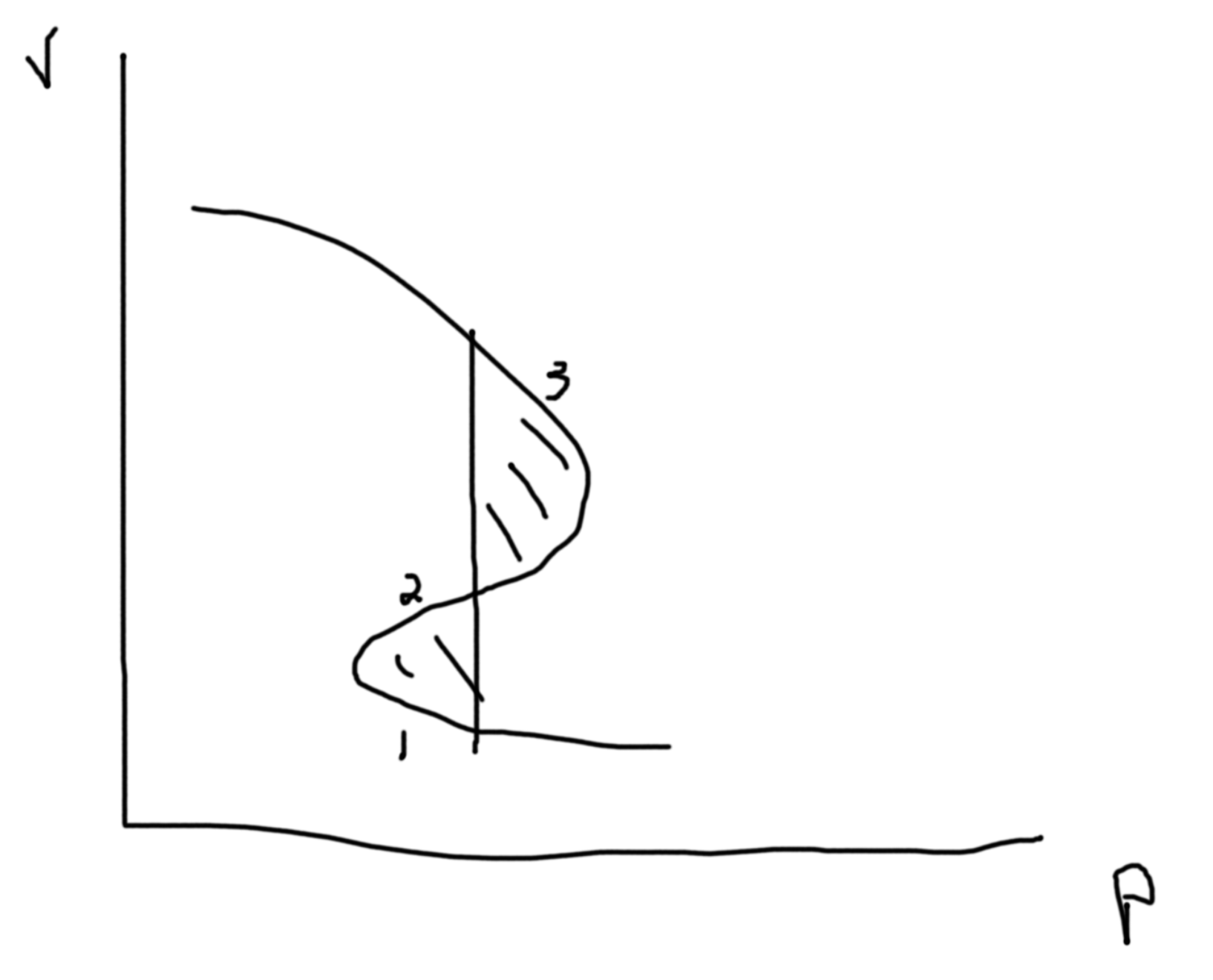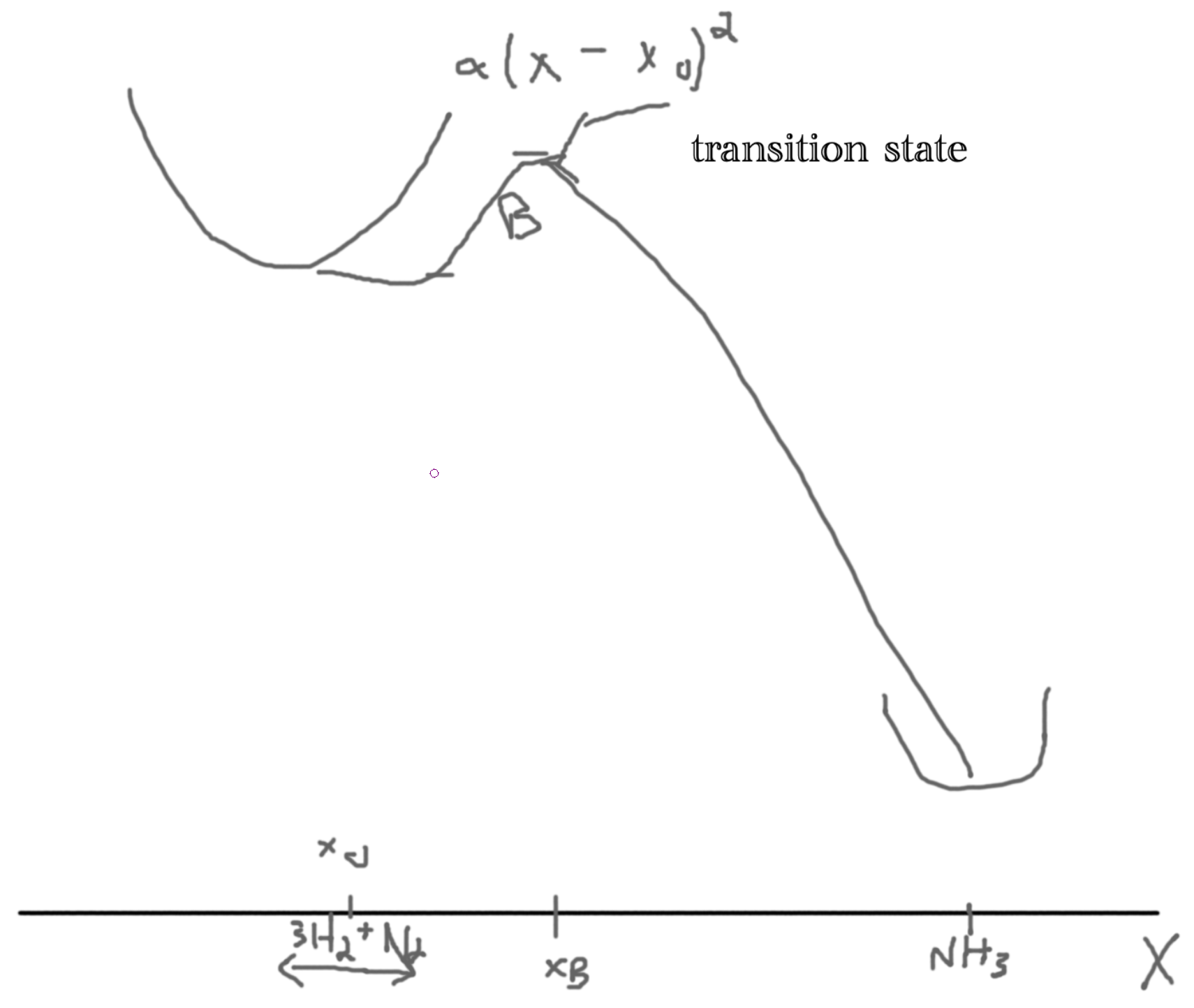# Gibbs Phase Rules

$$n_w$$: Number of ways to do work $$n_c$$: Number of chemical +1: Heating/ cooling $$N = n_w + n_c + 1$$ is the number of intensive parameters.

Constraints: If 2 phases coexist $$\mu_\ell = \mu_g$$ is one constraint. If 3 phases coexist $$\mu_\ell = \mu_g$$ and $$\mu_\ell=\mu_s$$ gives 2 constraints. So, let $$p$$ be the number of constraints. Then the degrees of freedom in our system is $$f = n_w + n_c + 1 - p$$. Suppose we have 1 species $$(n_c = 1)$$ and 1 way of doing work (mechanical/ pressure for example) so $$f = 3 - p$$. If we have 1 phase, $$p=1$$ so $$f=2$$ and so $$p(T)$$ is an area. If we have 2 phases, $$p=2$$ so $$f=1$$ and so $$p(T)$$ is a line. If we have 3 phases, $$p=3$$ so $$f=1$$ and so $$p(T)$$ is a point (triple point).

Say we fix $$N$$, then we can plot $$P$$ versus $$V/N$$.C.f. Suppose we have an alloy:Suppose that we have a constant temperature $$\to G(T,p,N)$$.

Then the Gibbs free energy of a liquid, we have a similar picture as $$F$$ with $$T$$ and $$N$$. So, plotting $$G(p,T,N)$$ versus temperature, we get two lines for liquids and gas that intersect at $$T_T$$. The liquid line has a more gradual slope and a lower intercept. In the region before the $$T_T$$ the gas line is called a supercooled gas and in the region after the liquid line (now above the gas line) is called a superheadted liquid.

So, $$G(T,p,N) = \mu N \Rightarrow \mu = \frac{G}{N}$$. I.e. the chemical potential of liquid is less than that of a gas. $$S = -\left(\frac{\partial G}{\partial T}\right)_{P,N}$$. $$\Delta S_{\ell-g} = \frac{Q}{T} = \frac{L N}{T}$$ where $$L$$ is the latent heat per particle. Hence, $$L = \frac{\Delta S \cdot T}{N}$$. IMPORTANT To compute the latent heat you need to compute the entropy change. $$\Delta S =$$ change in the slopes at the transition temperature.

## Van der Waals Equation of State

$$\left(p + a\frac{N^2}{V^2}\right)\left(V-Nb\right) = NkT$$ $$a$$ measures the attractive forces and $$b$$ measures the volume taken up by each particle.

Recall analysis from previous homeworks. $$p(V)\propto V^\gamma$$.

Stability conditions. For each conjugate pair you have (x, f) then $$\left(\frac{\partial x}{\partial f}\right)_{*}\geq 0$$. Except, $$\alpha = \frac{1}{V}\frac{\partial V}{\partial T}\geq \text{or} \leq 0$$. Compressibility gives condition for unstable states.

Between the two points we can draw a line at constant $$P$$ such that area above equals area below. This denotes area of equal coexistence and so $$\mu_\ell=\mu_g$$. The dotted lines are unphysical regions.From $$p\leftrightarrow G$$, $$dG = -SdT + Vdp + \mu dN$$. So $$\Delta G_{\ell-g} = \int_\ell^gdG = \int_{p_\ell}^{p_g}V(p)$$.### For a Reaction

$$3H_2+N_2 \rightleftharpoons 2NH_3$$. In this setup, these could all be in a gaseous state. Assume this is the case. So $$[H_2]^3[N_2]k_R(T) = \Gamma_R(T,p)$$ and $$\Gamma_L(T) = k_L(T)[NH_3]^2$$. Then, $$\frac{[NH_3]^2}{[H_2]^3[N_2]}=k_{eq}(T)$$. This is the Law of mass action. Finding $$k_{eq}(T)$$ we use the knowledge we are in gas phase and chemical potentials are equal.

Treat each constituent as an ideal gas. $$Z \to F \to \mu(T)$$. $$\Delta F = \left(\frac{\partial F}{\partial N_{H_2}}\right)\Delta N_{H_2} + \left(\frac{\partial F}{\partial N_{N_2}}\right)\Delta N_{N_2} + \left(\frac{\partial F}{\partial N_{NH_3}}\right)\Delta N_{NH_3}$$

Considering the rightwards direction: $$\Delta F_R = -3\mu_{H_2} -\mu_{N_2} +2\mu_{NH_3}$$. $$\Delta F_L = -\Delta F_R$$.

$$F(T,V,N) = NkT\left(\ln \frac{N}{V}\lambda^3 - 1\right)+NF_0$$ where the last term arises from the internal degrees of freedom of each of the $$N$$ particles. So, $$\mu(T,V,N) = \left(\frac{\partial F}{\partial N}\right)_{T,V} = kT\left(\ln\frac{N}{V}\lambda^3-1\right)+NkT\frac{1}{N}+F_0 = kT\ln \frac{N}{V} + kT\ln\lambda^3 + F_0 = kT\ln \frac{N}{V} + \mathbb{C}(T) + F_0$$. Inserting this in $$-3\mu_{H_2}-\mu_{N_2}+2\mu_{NH_{3}}=0$$, we get $$-3\ln[H_2]-\ln[N_2]+2\ln[NH_3] = 2\mathbb{C}_{H_2}+\mathbb{C}_{N_2}-2\mathbb{C}_{NH_3} + 3F_{0,H_2} + F_{0,N_2} - 2F_{0,NH_3} = -\Delta F_{net}$$. Then, $$\frac{[NH_3]^2}{[H_2]^3[N_2]} = k_{eq}(T) = k_0\exp\left(-\frac{\Delta F_{net}}{kT}\right)$$. Recall, $$\Delta F = \Delta E-T\Delta S \approx \Delta E_{net}$$ for solids and chemistry. Then, $$k_0 = \frac{\lambda_{H_2}^9\lambda_{N_2}^3}{\lambda_{NH_3}^6} = \frac{h^6 m_{NH_3}^3}{8\pi (k_BT)^3m_{H_2}^{9/2}m_{N_2}^{3/2}}\propto T^{-3}$$.

Aside: C.f. with hydrogen atom, $$\frac{[p][e]}{[H]}=k(T)$$ with $$n\cdot p=k(T)$$.

Consider the one way reaction consisting of 5 particles, $$3H_2 + N_2\to NH_3$$. If we have $$M$$ particles then we get a 3M dimensional space. Then we want to minimize energy in our 3M-1 dimensional space. Let $$X$$ be the reaction coordinate. Let the y-axis be the energy.We argue that the rate is proportional to the Boltzman factor, $$\gamma \propto \exp\left(-\frac{B}{kT}\right)$$, problem 6.11. The assumptions are: no recrossing, reactions are in local equilibrium (in ’well’), equal distribution determines the number of particles crossing the well. Let the probability distribution of the particles $$\rho(x)$$. Then the probability distribution at the bottom of the well is $$\rho(x_0)$$ and at the barrier $$\rho(x_b)$$ such that $$\frac{\rho(x_b)}{\rho(x_0)} = \frac{\exp(-U(x_B)/kT)}{\exp(-U(x))/kT} = \exp(-B/kT)$$. Since $$B\gg kT$$, most particles are near $$x_0$$. Then, $$U(x) = \frac{1}{2}M\omega^2(x-x_0)^2$$. So, $$\rho(x) = \sqrt{\frac{m\omega^2}{2\pi kT}}\exp\left(-\frac{m\omega^2}{2kT}(x-x_0)^2\right)$$ And so, $$\rho(x_0) = \sqrt{\frac{m\omega^2}{2\pi kT}}$$.

If the particle is $$\Delta x$$ away from the top of the barrier with velocity $$v$$ then $$\Delta x=v\Delta t$$ gives the rate $$\Gamma_V=\rho(x_B)v\frac{\Delta t}{\Delta t} = \rho(x_b)v$$. So, for all positive velocities, $$\Gamma = \int_0^\infty dv\Gamma_v\rho(v)$$. Then, we are in equilibrium our velocities are normal distributed velocities, $$\rho(v) \propto \exp(-E_{kin}/kT) = \exp(-\frac{Mv^2}{kT})$$ so $$\rho(v) = \sqrt{\frac{M}{2\pi kT}}\exp(-\frac{Mv^2}{2kT})$$. Thus, $$\Gamma = \rho(x_B)\int_0^\infty v\rho(v)dv = \frac{\omega}{2\pi}\exp\left(-\frac{B}{kT}\right)$$. Using multidimensional transition rate: $$\Gamma = \frac{\prod\limits_i\omega_i}{2\pi\prod\limits_j\omega_{j,B}}\exp\left(-\frac{B}{kT}\right)$$. Where $$\omega_{j,B}$$ denotes the saddle points.

If $$\omega$$ is large we get a steep well.

Thus, in general it is difficult to analyze kinetic behaviors from equilibrium treatments.

Created: 2023-06-25 Sun 02:27

Validate